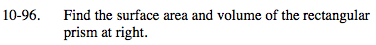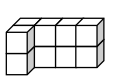### Home > MC2 > Chapter 10 > Lesson 10.2.2 > Problem10-96

10-96.Surface area is the total area of the surfaces of an object.
Volume is the space that an object occupies:
To find the volume, multiply the volume of one layer by the number of layers.

10 cubes make up this object.
How many sides act as a surface for the object and count towards the surface area?

Surface Area = 34 square units
Volume = 10 cubic units